What is LON-CAPA?  Who is LON-CAPA?  Documentation  Installation  Scholarship  Developers  Events

The LearningOnline Network with CAPA

Home > What is LON-CAPA? > ... > Examples of Individualizing Problems

Examples of Individualizing Problems

With LON-CAPA, an instructor can create and/or assemble individualized assignments, quizzes, and examinations with a large variety of conceptual questions and quantitative problems. "Individualized" means that each student sees a slightly different computer-generated problem (see demo problems). This encourages collaboration between students on a conceptual level, but prevents blind copying of answers.

Problems can include pictures, animations, graphics, tables, links, etc.. The writing and development is done through a web-based editor, and facilitated by templates.

Students are given instant feedback and relevant hints via the internet and may correct errors without penalty prior to an assignment's due date.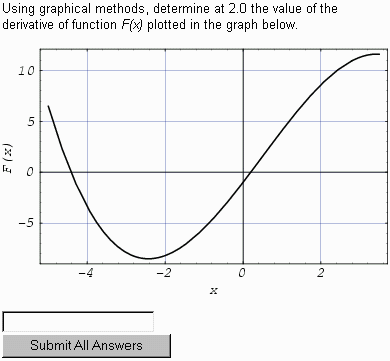LON-CAPA has dynamic plotting support - in this problem, both the plotted function and the x-value are different from student to student. The student has to graphically determine the slope.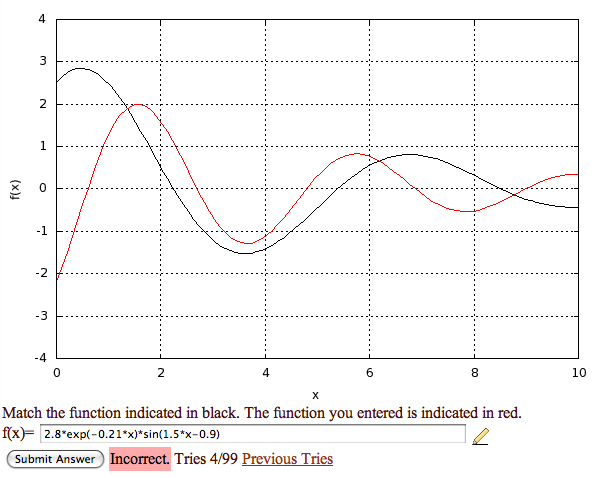Different students see different versions of a graph. In the example on the right, the student already entered an answer.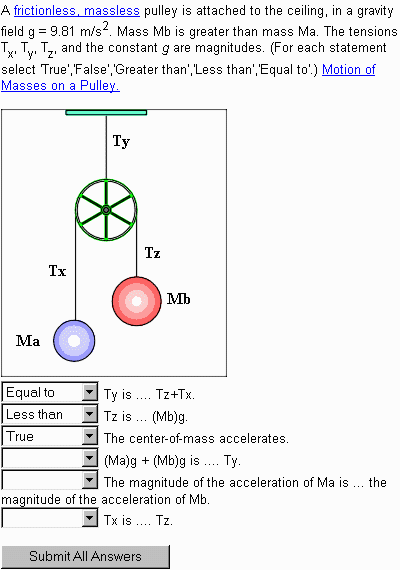The labels on the above image are different from student to student, so are the options and the order of the options. Labels on the image and in the options automatically correspond.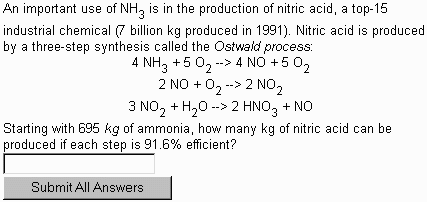LON-CAPA has full LaTeX-support from both online and print. In the example, the ammonia mass and the efficiency are randomized.LON-CAPA can accept and grade multi-dimensional symbolic answers, including mathematical equivalence. In these examples, the functions in the vector are randomized.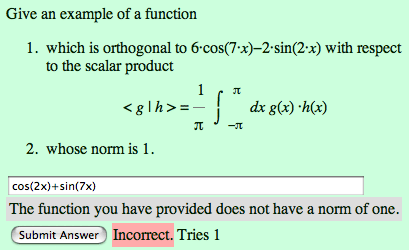LON-CAPA can check for algebraic properties. In this example the function that the student enters is integrated using the underlying computer algebra system.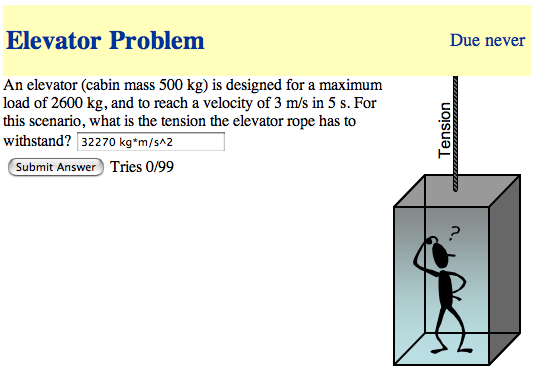LON-CAPA has full support for physical units, understands all SI-units and their relationships, as well as all prefixes.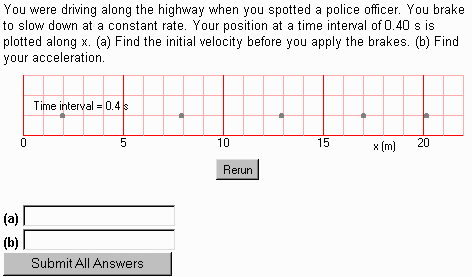In this example, the student can see the plot build up over time. The parameters for this plotting applet are randomized. There are multiple answer fields, which require physical units in the answer.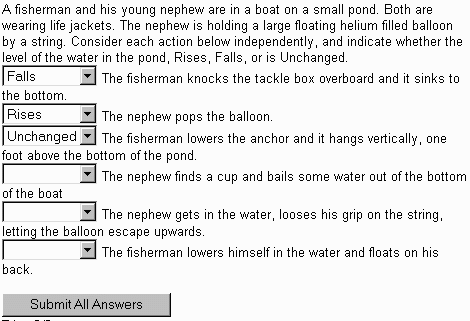In this example, the options and the order of the options are randomized.In this example, the options are randomized.Tolerances for numerical answers can be set symmetric and asymmetric, absolute and relative. In addition, ranges for number of significant digits can be specified.LON-CAPA supports input and grading of chemical structures.